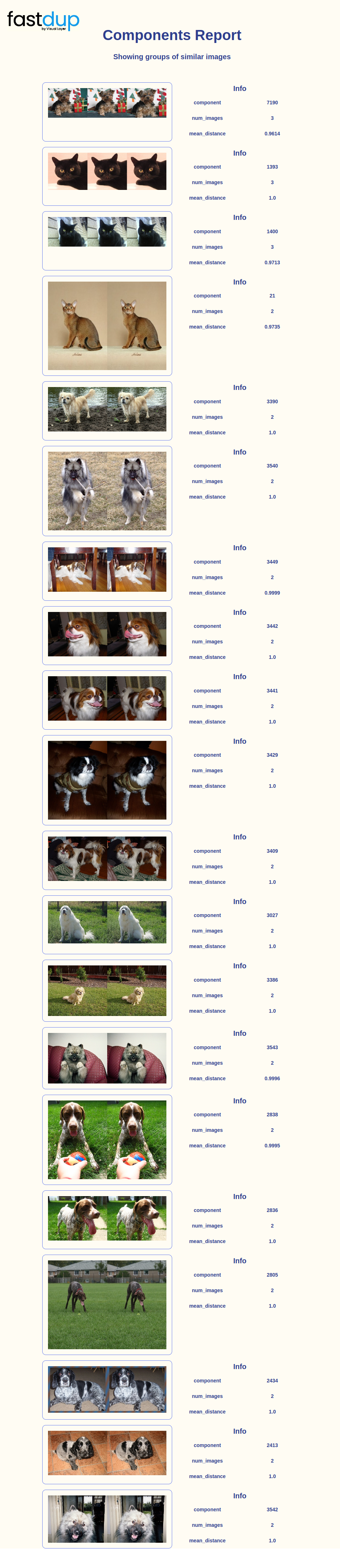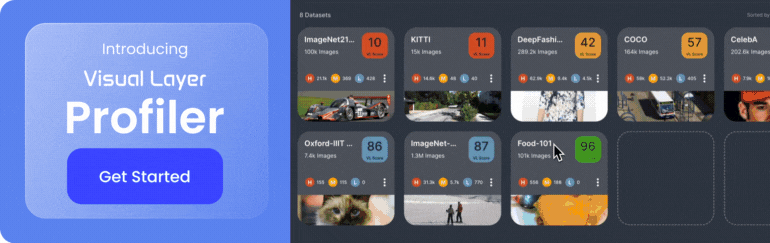# Run On Pre-computed Feature Vectors

If you have existing feature vectors, you can run fastdup over the feature vectors to analyze for issues.

If you have pre-computed feature vectors using fastdup or any other methods, you can analyze for issues in your dataset by inputting the features into fastdup directly. This drastically reduces the run time.

The following is a code snippet to run with your own feature stored in a `numpy` matrix, along with a list of the matching filenames.

``````import numpy as np
import fastdup

# Replace the below code with computation of your own features
matrix = np.random.rand(2, 576).astype('float32')
flist = ["/data/myimage1.jpg", "/data/myimage2.jpg"]

# Files should contain absolute path and not relative path
fd = fastdup.create(input_dir='/data/', work_dir='output')
fd.run(annotations=flist, embeddings=matrix)
``````
``````import fastdup
import numpy as np
import os
input_dir = '/Users/dannybickson/visual_database/cxx/unittests/two_images/'
flist = os.listdir(input_dir)
flist = [os.path.join(input_dir, f) for f in flist]

# replace the below code with computation of your own features
matrix = np.random.rand(2, 576).astype('float32')

# save the embedding along the filenames into a working folder
!mkdir -p embedding_input
fastdup.save_binary_feature('embedding_input', flist, matrix)
fastdup.run('~/visual_database/cxx/unittests/two_images/', run_mode=2, work_dir='embedding_input')
``````

# End-to-end Example

This section shows an end-to-end example of using pre-computed feature vectors using DINOv2 and using the features in a fastdup run.

## Step 1: Compute the feature vectors using DINOv2 model in fastdup.

``````import fastdup

fd = fastdup.create(input_dir="images/", work_dir='work_dir')
fd.run(model_path='dinov2s')
``````

👍

Tip

Try out our DINOv2 example on Colab/Kaggle and pre-compute the feature vectors of your dataset.

Or use fastdup to compute the feature vectors with your own ONNX model.

## Step 2: Load the pre-computed feature vectors.

Upon completion of the run, fastdup saves the feature vectors locally in the`work_dir/atrain_features.dat`file.

``````filenames, feature_vec = fastdup.load_binary_feature("work_dir/atrain_features.dat",
d=384)
``````

Inspect the dimension of the feature vectors.

``````feature_vec.shape
``````
``````(7384, 384)
``````

📘

Note

• `7384` corresponds to the number of images in the dataset.
• `384`corresponds to the output dimension of the DINOv2s model.

## Step 3: Run fastdup.

To run fastdup on the pre-computed feature vectors, point the `annotations` parameter to the filenames and `embeddings` parameter to the feature vector.

``````fd = fastdup.create(input_dir="images/", work_dir='output')
fd.run(annotations=filenames, embeddings=feature_vec)
``````

👍

Tip

The benefit of running fastdup over pre-computed feature vector is speed. Compared to running fastdup on the raw images, running fastdup over pre-computed features takes only a fraction of the time otherwise.

The time it takes to run the above code is approximately 15s.

## Step 4: View Galleries.

You can use all of fastdup gallery methods to view duplicates, clusters, etc.

``````fd.vis.component_gallery()
``````## Step 5: Iterate

Let's suppose you are not satisfied with the image cluster results above, you can always tweak the `run` parameters until a desired outcome is reached.

For example, let's rerun fastdup with `ccthreshold=0.8`and visualize the clusters again.

``````fd.run(annotations=filenames, embeddings=feature_vec, ccthreshold=0.8)
``````

👍

Tip

Read more on how to tune the run parameters to obtain a desired output on your dataset here.

# Wrap Up

In this tutorial, we showed how you can run fastdup using pre-computed feature vectors. Running over pre-computed feature vectors significantly reduces run time compared to running over raw image files.

# VL Profiler - A faster and easier way to diagnose and visualize dataset issues

The team behind fastdup also recently launched VL Profiler, a no-code cloud-based platform that lets you leverage fastdup in the browser.

VL Profiler lets you find:

• Duplicates/near-duplicates.
• Outliers.
• Mislabels.
• Non-useful images.

Here's a highlight of the issues found in the RVL-CDIP test dataset on the VL Profiler.

👍

Free Usage